# 002-functions-and-circuits.ppt

31 de May de 2023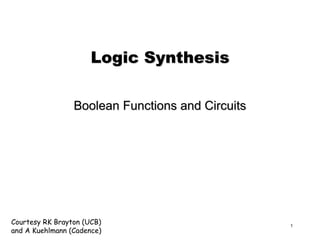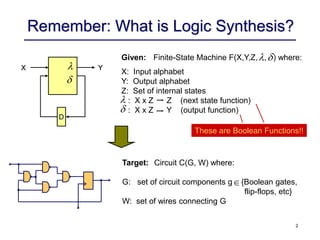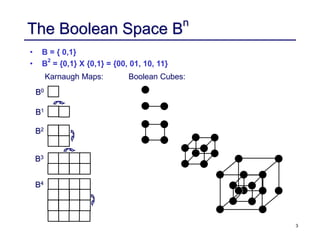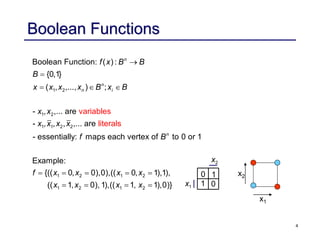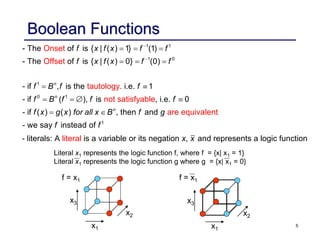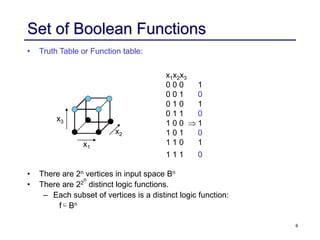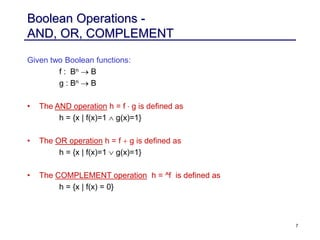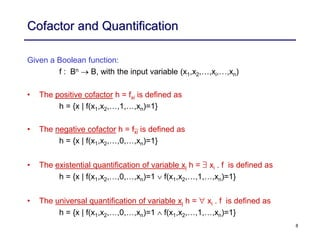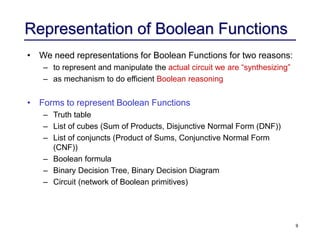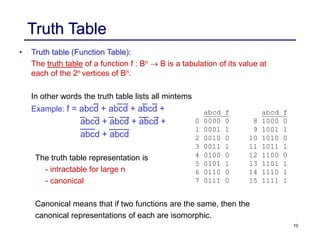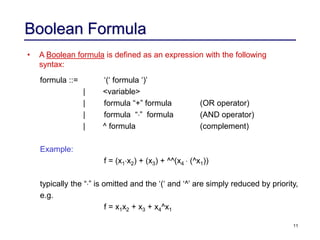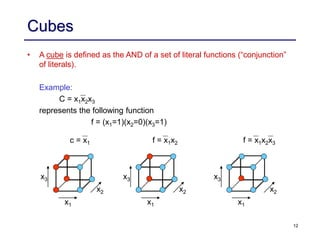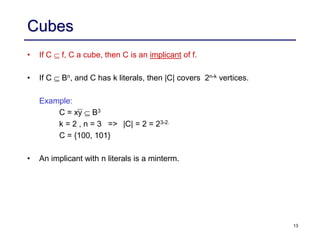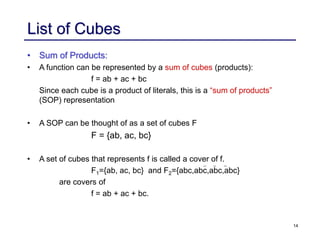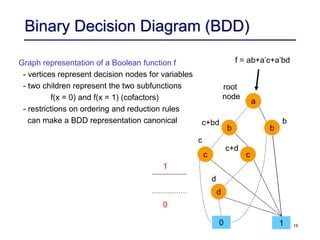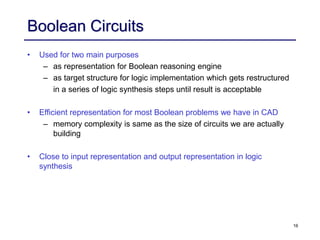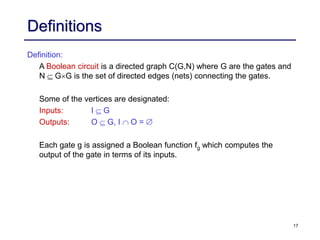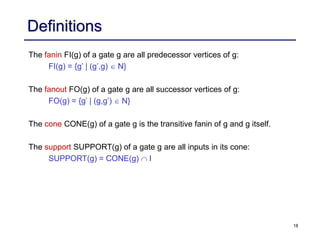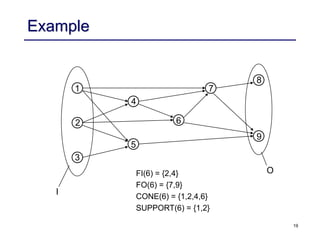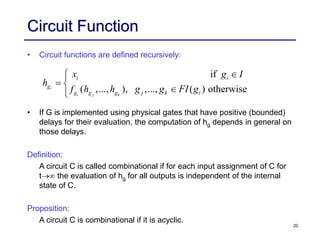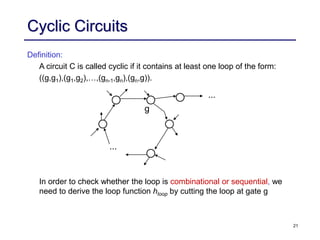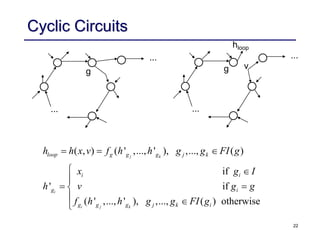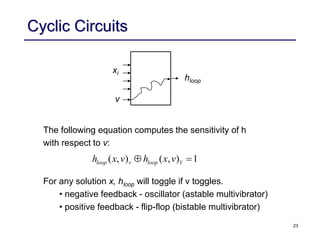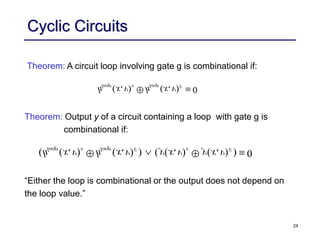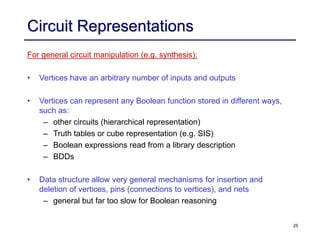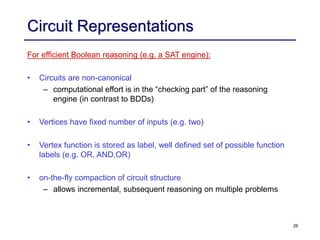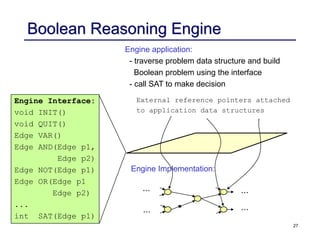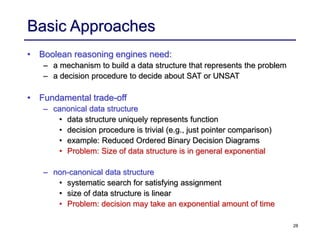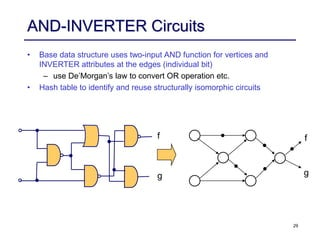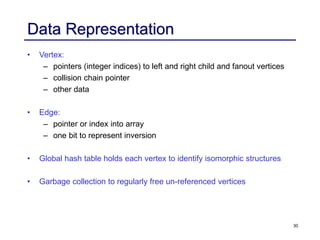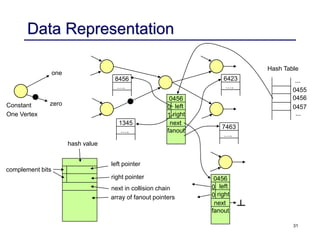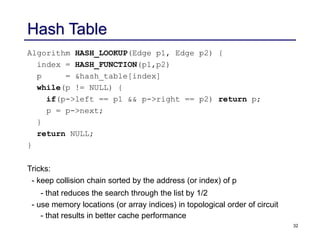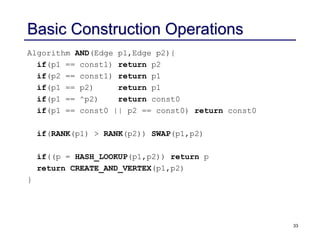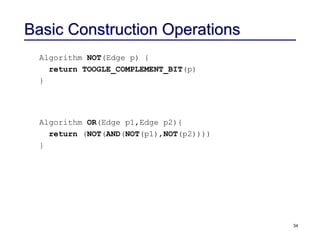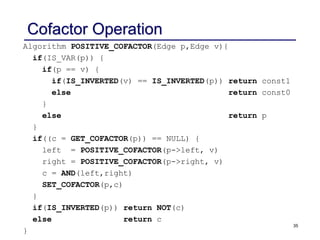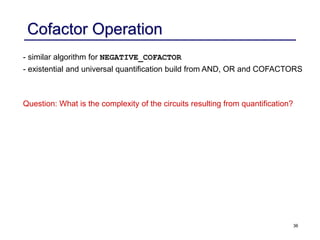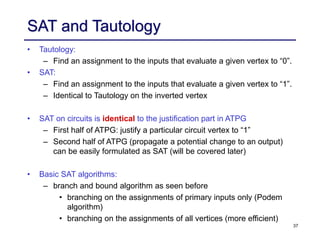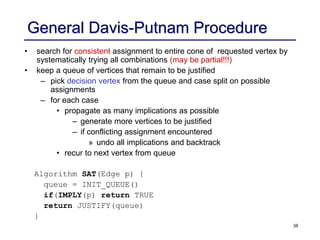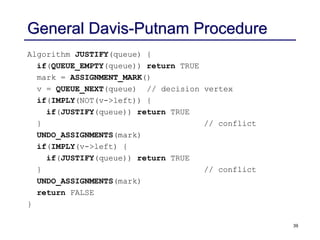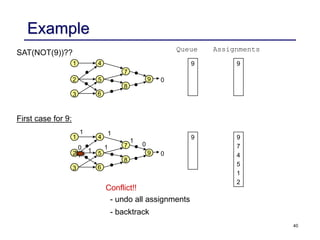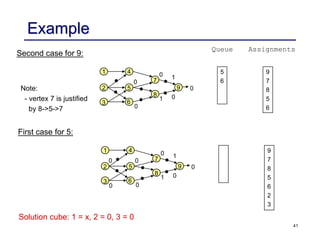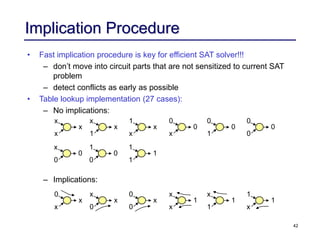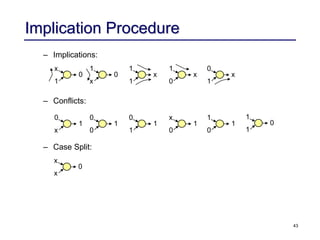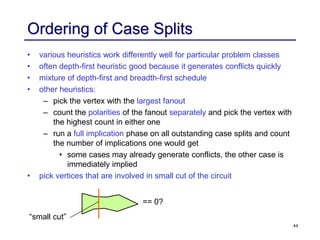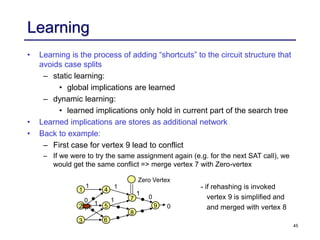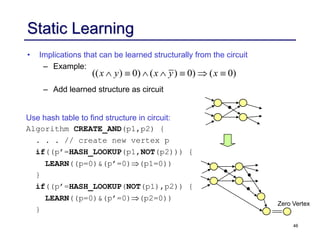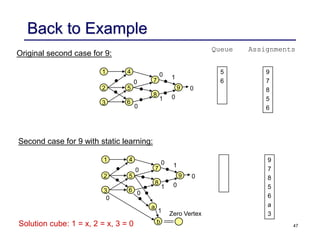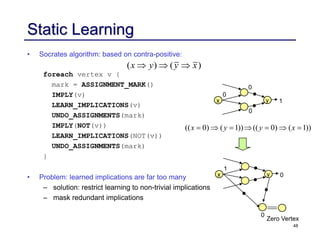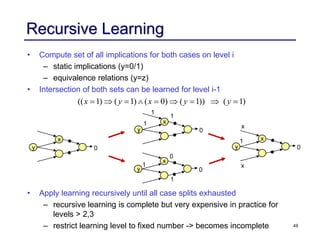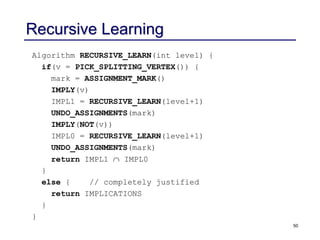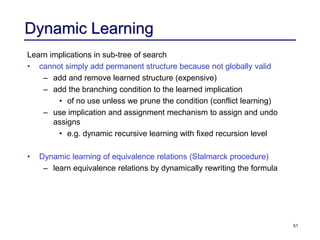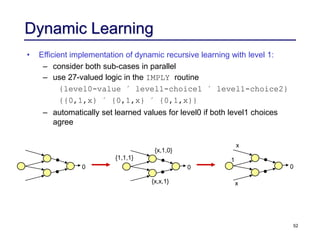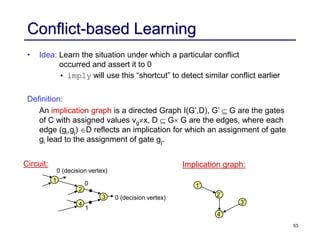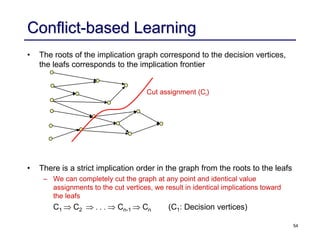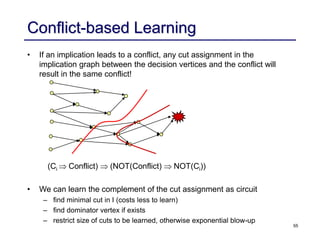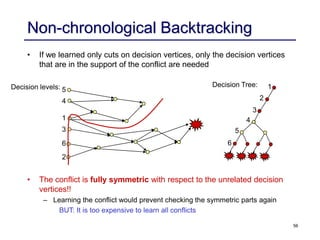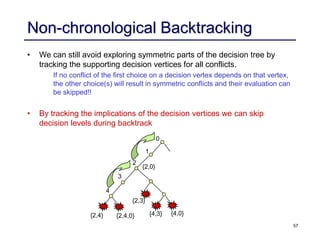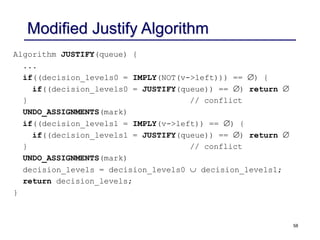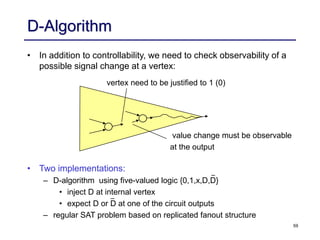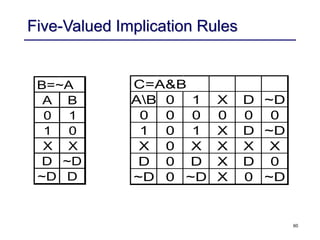1 de 60

### 002-functions-and-circuits.ppt

• 1. Courtesy RK Brayton (UCB) and A Kuehlmann (Cadence) 1 Logic Synthesis Boolean Functions and Circuits
• 2. 2 Remember: What is Logic Synthesis? Given: Finite-State Machine F(X,Y,Z, , ) where: D X Y     X: Input alphabet Y: Output alphabet Z: Set of internal states : X x Z Z (next state function) : X x Z Y (output function)   Target: Circuit C(G, W) where: G: set of circuit components g {Boolean gates, flip-flops, etc} W: set of wires connecting G  These are Boolean Functions!!
• 3. 3 The Boolean Space B n • B = { 0,1} • B 2 = {0,1} X {0,1} = {00, 01, 10, 11} B0 B1 B2 B3 B4 Karnaugh Maps: Boolean Cubes:
• 4. 4 Boolean Functions           1 2 1 2 1 1 2 2 1 2 1 2 Boolean Function: ( ) : {0,1} ( , ,..., ) ; - , ,... are - , , , ,... are - essentially: maps each vertex of to 0 or 1 Exampl vari e: {(( 0, 0),0),(( 0, ables literals 1 n n n i n f x B B B x x x x B x B x x x x x x f B f x x x x     1 2 1 2 ),1), (( 1, 0), 1),(( 1, 1),0)} x x x x 1 x 2 x 0 0 1 1 x2 x1
• 5. 5 Boolean Functions                 1 1 1 0 1 0 1 - The of is { | ( ) 1} (1) - The of is { | ( ) 0} (0) - if , is the i.e. 1 - if ( ), is Onset Offset tautology. not satisfyabl , i.e. 0 - if ( ) ( ) , t e hen a n n n f x f x f f f x f x f f f B f f f B f f f f x g x for all x B f 1 nd - we say are equiva instea lent d of g f f - literals: A is a variable or its negation , and represents a logic l function iteral x x Literal x1 represents the logic function f, where f = {x| x1 = 1} Literal x1 represents the logic function g where g = {x| x1 = 0} x3 x1 x2 x1 x2 x3 f = x1 f = x1
• 6. 6 Set of Boolean Functions • There are 2n vertices in input space Bn • There are 22 n distinct logic functions. – Each subset of vertices is a distinct logic function: f  Bn x1x2x3 0 0 0 1 0 0 1 0 0 1 0 1 0 1 1 0 1 0 0  1 1 0 1 0 1 1 0 1 1 1 1 0 x1 x2 x3 • Truth Table or Function table:
• 7. 7 Boolean Operations - AND, OR, COMPLEMENT Given two Boolean functions: f : Bn  B g : Bn  B • The AND operation h = f  g is defined as h = {x | f(x)=1  g(x)=1} • The OR operation h = f + g is defined as h = {x | f(x)=1  g(x)=1} • The COMPLEMENT operation h = ^f is defined as h = {x | f(x) = 0}
• 8. 8 Cofactor and Quantification Given a Boolean function: f : Bn  B, with the input variable (x1,x2,…,xi,…,xn) • The positive cofactor h = fxi is defined as h = {x | f(x1,x2,…,1,…,xn)=1} • The negative cofactor h = fxi is defined as h = {x | f(x1,x2,…,0,…,xn)=1} • The existential quantification of variable xi h = \$ xi . f is defined as h = {x | f(x1,x2,…,0,…,xn)=1 f(x1,x2,…,1,…,xn)=1} • The universal quantification of variable xi h = " xi . f is defined as h = {x | f(x1,x2,…,0,…,xn)=1 f(x1,x2,…,1,…,xn)=1}
• 9. 9 Representation of Boolean Functions • We need representations for Boolean Functions for two reasons: – to represent and manipulate the actual circuit we are “synthesizing” – as mechanism to do efficient Boolean reasoning • Forms to represent Boolean Functions – Truth table – List of cubes (Sum of Products, Disjunctive Normal Form (DNF)) – List of conjuncts (Product of Sums, Conjunctive Normal Form (CNF)) – Boolean formula – Binary Decision Tree, Binary Decision Diagram – Circuit (network of Boolean primitives)
• 10. 10 Truth Table • Truth table (Function Table): The truth table of a function f : Bn  B is a tabulation of its value at each of the 2n vertices of Bn. In other words the truth table lists all mintems Example: f = abcd + abcd + abcd + abcd + abcd + abcd + abcd + abcd The truth table representation is - intractable for large n - canonical Canonical means that if two functions are the same, then the canonical representations of each are isomorphic. abcd f 0 0000 0 1 0001 1 2 0010 0 3 0011 1 4 0100 0 5 0101 1 6 0110 0 7 0111 0 abcd f 8 1000 0 9 1001 1 10 1010 0 11 1011 1 12 1100 0 13 1101 1 14 1110 1 15 1111 1
• 11. 11 Boolean Formula • A Boolean formula is defined as an expression with the following syntax: formula ::= ‘(‘ formula ‘)’ | <variable> | formula “+” formula (OR operator) | formula “” formula (AND operator) | ^ formula (complement) Example: f = (x1x2) + (x3) + ^^(x4  (^x1)) typically the “” is omitted and the ‘(‘ and ‘^’ are simply reduced by priority, e.g. f = x1x2 + x3 + x4^x1
• 12. 12 Cubes • A cube is defined as the AND of a set of literal functions (“conjunction” of literals). Example: C = x1x2x3 represents the following function f = (x1=1)(x2=0)(x3=1) x1 x2 x3 c = x1 x1 x2 x3 f = x1x2 x1 x2 x3 f = x1x2x3
• 13. 13 Cubes • If C  f, C a cube, then C is an implicant of f. • If C  Bn, and C has k literals, then |C| covers 2n-k vertices. Example: C = xy  B3 k = 2 , n = 3 => |C| = 2 = 23-2. C = {100, 101} • An implicant with n literals is a minterm.
• 14. 14 List of Cubes • Sum of Products: • A function can be represented by a sum of cubes (products): f = ab + ac + bc Since each cube is a product of literals, this is a “sum of products” (SOP) representation • A SOP can be thought of as a set of cubes F F = {ab, ac, bc} • A set of cubes that represents f is called a cover of f. F1={ab, ac, bc} and F2={abc,abc,abc,abc} are covers of f = ab + ac + bc.
• 15. 15 Binary Decision Diagram (BDD) f = ab+a’c+a’bd 1 0 c a b b c c d 0 1 c+bd b root node c+d d Graph representation of a Boolean function f - vertices represent decision nodes for variables - two children represent the two subfunctions f(x = 0) and f(x = 1) (cofactors) - restrictions on ordering and reduction rules can make a BDD representation canonical
• 16. 16 Boolean Circuits • Used for two main purposes – as representation for Boolean reasoning engine – as target structure for logic implementation which gets restructured in a series of logic synthesis steps until result is acceptable • Efficient representation for most Boolean problems we have in CAD – memory complexity is same as the size of circuits we are actually building • Close to input representation and output representation in logic synthesis
• 17. 17 Definitions Definition: A Boolean circuit is a directed graph C(G,N) where G are the gates and N GG is the set of directed edges (nets) connecting the gates. Some of the vertices are designated: Inputs: I G Outputs: O G, I O =  Each gate g is assigned a Boolean function fg which computes the output of the gate in terms of its inputs.
• 18. 18 Definitions The fanin FI(g) of a gate g are all predecessor vertices of g: FI(g) = {g’ | (g’,g) N} The fanout FO(g) of a gate g are all successor vertices of g: FO(g) = {g’ | (g,g’) N} The cone CONE(g) of a gate g is the transitive fanin of g and g itself. The support SUPPORT(g) of a gate g are all inputs in its cone: SUPPORT(g) = CONE(g) I
• 19. 19 Example I O 6 FI(6) = {2,4} FO(6) = {7,9} CONE(6) = {1,2,4,6} SUPPORT(6) = {1,2} 1 5 3 4 7 8 9 2
• 20. 20 Circuit Function • Circuit functions are defined recursively: • If G is implemented using physical gates that have positive (bounded) delays for their evaluation, the computation of hg depends in general on those delays. Definition: A circuit C is called combinational if for each input assignment of C for t the evaluation of hg for all outputs is independent of the internal state of C. Proposition: A circuit C is combinational if it is acyclic. if ( ,..., ), ,..., ( ) otherwise i i j k i i g g g g j k i x g I h f h h g g FI g        
• 21. 21 Cyclic Circuits Definition: A circuit C is called cyclic if it contains at least one loop of the form: ((g,g1),(g1,g2),…,(gn-1,gn),(gn,g)). In order to check whether the loop is combinational or sequential, we need to derive the loop function hloop by cutting the loop at gate g ... ... g
• 22. 22 Cyclic Circuits ... ... ... v g g hloop ( , ) ( ' ,..., ' ), ,..., ( ) if ' if ( ' ,..., ' ), j k i i j k loop g g g j k i i g i g g g h h x v f h h g g FI g x g I h v g g f h h g       ,..., ( ) otherwise j k i g FI g       ...
• 23. 23 Cyclic Circuits ( , ) ( , ) 1 loop v loop v h x v h x v   hloop xi v The following equation computes the sensitivity of h with respect to v: For any solution x, hloop will toggle if v toggles. • negative feedback - oscillator (astable multivibrator) • positive feedback - flip-flop (bistable multivibrator)
• 24. 24 Cyclic Circuits ( , ) ( , ) 0 loop v loop v h x v h x v   Theorem: A circuit loop involving gate g is combinational if: ( ( , ) ( , ) ) ( ( , ) ( , ) ) 0 loop v loop v v v h x v h x v y x v y x v     Theorem: Output y of a circuit containing a loop with gate g is combinational if: “Either the loop is combinational or the output does not depend on the loop value.”
• 25. 25 Circuit Representations For general circuit manipulation (e.g. synthesis): • Vertices have an arbitrary number of inputs and outputs • Vertices can represent any Boolean function stored in different ways, such as: – other circuits (hierarchical representation) – Truth tables or cube representation (e.g. SIS) – Boolean expressions read from a library description – BDDs • Data structure allow very general mechanisms for insertion and deletion of vertices, pins (connections to vertices), and nets – general but far too slow for Boolean reasoning
• 26. 26 Circuit Representations For efficient Boolean reasoning (e.g. a SAT engine): • Circuits are non-canonical – computational effort is in the “checking part” of the reasoning engine (in contrast to BDDs) • Vertices have fixed number of inputs (e.g. two) • Vertex function is stored as label, well defined set of possible function labels (e.g. OR, AND,OR) • on-the-fly compaction of circuit structure – allows incremental, subsequent reasoning on multiple problems
• 27. 27 Boolean Reasoning Engine Engine Interface: void INIT() void QUIT() Edge VAR() Edge AND(Edge p1, Edge p2) Edge NOT(Edge p1) Edge OR(Edge p1 Edge p2) ... int SAT(Edge p1) Engine application: - traverse problem data structure and build Boolean problem using the interface - call SAT to make decision Engine Implementation: ... ... ... ... External reference pointers attached to application data structures
• 28. 28 Basic Approaches • Boolean reasoning engines need: – a mechanism to build a data structure that represents the problem – a decision procedure to decide about SAT or UNSAT • Fundamental trade-off – canonical data structure • data structure uniquely represents function • decision procedure is trivial (e.g., just pointer comparison) • example: Reduced Ordered Binary Decision Diagrams • Problem: Size of data structure is in general exponential – non-canonical data structure • systematic search for satisfying assignment • size of data structure is linear • Problem: decision may take an exponential amount of time
• 29. 29 AND-INVERTER Circuits • Base data structure uses two-input AND function for vertices and INVERTER attributes at the edges (individual bit) – use De’Morgan’s law to convert OR operation etc. • Hash table to identify and reuse structurally isomorphic circuits f g g f
• 30. 30 Data Representation • Vertex: – pointers (integer indices) to left and right child and fanout vertices – collision chain pointer – other data • Edge: – pointer or index into array – one bit to represent inversion • Global hash table holds each vertex to identify isomorphic structures • Garbage collection to regularly free un-referenced vertices
• 31. 31 Data Representation 0456 left right next fanout 1345 …. 8456 …. 6423 …. 7463 …. 0 1 hash value left pointer right pointer next in collision chain array of fanout pointers complement bits Constant One Vertex zero one 0456 0455 0457 ... ... Hash Table 0456 left right next fanout 0 0
• 32. 32 Hash Table Algorithm HASH_LOOKUP(Edge p1, Edge p2) { index = HASH_FUNCTION(p1,p2) p = &hash_table[index] while(p != NULL) { if(p->left == p1 && p->right == p2) return p; p = p->next; } return NULL; } Tricks: - keep collision chain sorted by the address (or index) of p - that reduces the search through the list by 1/2 - use memory locations (or array indices) in topological order of circuit - that results in better cache performance
• 33. 33 Basic Construction Operations Algorithm AND(Edge p1,Edge p2){ if(p1 == const1) return p2 if(p2 == const1) return p1 if(p1 == p2) return p1 if(p1 == ^p2) return const0 if(p1 == const0 || p2 == const0) return const0 if(RANK(p1) > RANK(p2)) SWAP(p1,p2) if((p = HASH_LOOKUP(p1,p2)) return p return CREATE_AND_VERTEX(p1,p2) }
• 34. 34 Basic Construction Operations Algorithm OR(Edge p1,Edge p2){ return (NOT(AND(NOT(p1),NOT(p2)))) } Algorithm NOT(Edge p) { return TOOGLE_COMPLEMENT_BIT(p) }
• 35. 35 Cofactor Operation Algorithm POSITIVE_COFACTOR(Edge p,Edge v){ if(IS_VAR(p)) { if(p == v) { if(IS_INVERTED(v) == IS_INVERTED(p)) return const1 else return const0 } else return p } if((c = GET_COFACTOR(p)) == NULL) { left = POSITIVE_COFACTOR(p->left, v) right = POSITIVE_COFACTOR(p->right, v) c = AND(left,right) SET_COFACTOR(p,c) } if(IS_INVERTED(p)) return NOT(c) else return c }
• 36. 36 Cofactor Operation - similar algorithm for NEGATIVE_COFACTOR - existential and universal quantification build from AND, OR and COFACTORS Question: What is the complexity of the circuits resulting from quantification?
• 37. 37 SAT and Tautology • Tautology: – Find an assignment to the inputs that evaluate a given vertex to “0”. • SAT: – Find an assignment to the inputs that evaluate a given vertex to “1”. – Identical to Tautology on the inverted vertex • SAT on circuits is identical to the justification part in ATPG – First half of ATPG: justify a particular circuit vertex to “1” – Second half of ATPG (propagate a potential change to an output) can be easily formulated as SAT (will be covered later) • Basic SAT algorithms: – branch and bound algorithm as seen before • branching on the assignments of primary inputs only (Podem algorithm) • branching on the assignments of all vertices (more efficient)
• 38. 38 General Davis-Putnam Procedure • search for consistent assignment to entire cone of requested vertex by systematically trying all combinations (may be partial!!!) • keep a queue of vertices that remain to be justified – pick decision vertex from the queue and case split on possible assignments – for each case • propagate as many implications as possible – generate more vertices to be justified – if conflicting assignment encountered » undo all implications and backtrack • recur to next vertex from queue Algorithm SAT(Edge p) { queue = INIT_QUEUE() if(IMPLY(p) return TRUE return JUSTIFY(queue) }
• 39. 39 General Davis-Putnam Procedure Algorithm JUSTIFY(queue) { if(QUEUE_EMPTY(queue)) return TRUE mark = ASSIGNMENT_MARK() v = QUEUE_NEXT(queue) // decision vertex if(IMPLY(NOT(v->left)) { if(JUSTIFY(queue)) return TRUE } // conflict UNDO_ASSIGNMENTS(mark) if(IMPLY(v->left) { if(JUSTIFY(queue)) return TRUE } // conflict UNDO_ASSIGNMENTS(mark) return FALSE }
• 40. 40 Example First case for 9: Queue Assignments 1 6 2 5 8 7 3 4 9 9 0 1 6 2 5 8 7 3 4 9 9 0 9 9 7 4 5 1 2 0 1 1 1 1 0 1 Conflict!! - undo all assignments - backtrack SAT(NOT(9))??
• 41. 41 Example Second case for 9: First case for 5: Assignments 1 6 2 5 8 7 3 4 9 5 6 0 9 7 8 5 6 0 1 0 0 0 1 Queue 1 6 2 5 8 7 3 4 9 0 9 7 8 5 6 2 3 0 1 0 0 0 1 0 Note: - vertex 7 is justified by 8->5->7 0 Solution cube: 1 = x, 2 = 0, 3 = 0
• 42. 42 Implication Procedure • Fast implication procedure is key for efficient SAT solver!!! – don’t move into circuit parts that are not sensitized to current SAT problem – detect conflicts as early as possible • Table lookup implementation (27 cases): – No implications: – Implications: x x x x 1 x 1 x x 0 x 0 0 1 0 0 0 0 x 0 0 1 0 0 1 1 1 0 x x x 0 x 0 0 x x x 1 x 1 1 1 x 1
• 43. 43 Implication Procedure – Implications: – Conflicts: – Case Split: x 1 0 1 x 0 1 1 x 1 0 x 0 1 x 1 1 0 x x 0 0 x 1 0 0 1 0 1 1 x 0 1 1 0 1
• 44. 44 Ordering of Case Splits • various heuristics work differently well for particular problem classes • often depth-first heuristic good because it generates conflicts quickly • mixture of depth-first and breadth-first schedule • other heuristics: – pick the vertex with the largest fanout – count the polarities of the fanout separately and pick the vertex with the highest count in either one – run a full implication phase on all outstanding case splits and count the number of implications one would get • some cases may already generate conflicts, the other case is immediately implied • pick vertices that are involved in small cut of the circuit == 0? “small cut”
• 45. 45 Learning • Learning is the process of adding “shortcuts” to the circuit structure that avoids case splits – static learning: • global implications are learned – dynamic learning: • learned implications only hold in current part of the search tree • Learned implications are stores as additional network • Back to example: – First case for vertex 9 lead to conflict – If we were to try the same assignment again (e.g. for the next SAT call), we would get the same conflict => merge vertex 7 with Zero-vertex 1 6 2 5 8 7 3 4 9 0 0 1 1 1 1 0 1 Zero Vertex - if rehashing is invoked vertex 9 is simplified and and merged with vertex 8
• 46. 46 Static Learning • Implications that can be learned structurally from the circuit – Example: – Add learned structure as circuit (( ) 0) ( ) 0) ( 0) x y x y x        Zero Vertex Use hash table to find structure in circuit: Algorithm CREATE_AND(p1,p2) { . . . // create new vertex p if((p’=HASH_LOOKUP(p1,NOT(p2))) { LEARN((p=0)&(p’=0)(p1=0)) } if((p’=HASH_LOOKUP(NOT(p1),p2)) { LEARN((p=0)&(p’=0)(p2=0)) }
• 47. 47 Back to Example Original second case for 9: Assignments 1 6 2 5 8 7 3 4 9 5 6 0 9 7 8 5 6 0 1 0 0 0 1 Queue Second case for 9 with static learning: 1 6 2 5 8 7 3 4 9 0 9 7 8 5 6 a 3 0 1 0 0 0 1 Zero Vertex a b 1 0 Solution cube: 1 = x, 2 = x, 3 = 0
• 48. 48 Static Learning • Socrates algorithm: based on contra-positive: foreach vertex v { mark = ASSIGNMENT_MARK() IMPLY(v) LEARN_IMPLICATIONS(v) UNDO_ASSIGNMENTS(mark) IMPLY(NOT(v)) LEARN_IMPLICATIONS(NOT(v)) UNDO_ASSIGNMENTS(mark) } • Problem: learned implications are far too many – solution: restrict learning to non-trivial implications – mask redundant implications ( ) ( ) x y y x    x y 1 0 0 0 (( 0) ( 1)) (( 0) ( 1)) x y y x        x y 0 1 Zero Vertex 0 0
• 49. 49 Recursive Learning • Compute set of all implications for both cases on level i – static implications (y=0/1) – equivalence relations (y=z) • Intersection of both sets can be learned for level i-1 • Apply learning recursively until all case splits exhausted – recursive learning is complete but very expensive in practice for levels > 2,3 – restrict learning level to fixed number -> becomes incomplete (( 1) ( 1) ( 0) ( 1)) ( 1) x y x y y          x y 0 x y 0 x y 0 1 0 1 1 1 1 x y 0 1 x x
• 50. 50 Recursive Learning Algorithm RECURSIVE_LEARN(int level) { if(v = PICK_SPLITTING_VERTEX()) { mark = ASSIGNMENT_MARK() IMPLY(v) IMPL1 = RECURSIVE_LEARN(level+1) UNDO_ASSIGNMENTS(mark) IMPLY(NOT(v)) IMPL0 = RECURSIVE_LEARN(level+1) UNDO_ASSIGNMENTS(mark) return IMPL1  IMPL0 } else { // completely justified return IMPLICATIONS } }
• 51. 51 Dynamic Learning Learn implications in sub-tree of search • cannot simply add permanent structure because not globally valid – add and remove learned structure (expensive) – add the branching condition to the learned implication • of no use unless we prune the condition (conflict learning) – use implication and assignment mechanism to assign and undo assigns • e.g. dynamic recursive learning with fixed recursion level • Dynamic learning of equivalence relations (Stalmarck procedure) – learn equivalence relations by dynamically rewriting the formula
• 52. 52 Dynamic Learning • Efficient implementation of dynamic recursive learning with level 1: – consider both sub-cases in parallel – use 27-valued logic in the IMPLY routine {level0-value ´ level1-choice1 ´ level1-choice2} {{0,1,x} ´ {0,1,x} ´ {0,1,x}} – automatically set learned values for level0 if both level1 choices agree 0 0 0 {x,1,0} {x,x,1} {1,1,1} 1 x x
• 53. 53 Conflict-based Learning • Idea: Learn the situation under which a particular conflict occurred and assert it to 0 • imply will use this “shortcut” to detect similar conflict earlier Definition: An implication graph is a directed Graph I(G’,D), G’ G are the gates of C with assigned values vgx, D  G G are the edges, where each edge (gi,gj) D reflects an implication for which an assignment of gate gi lead to the assignment of gate gj. 0 (decision vertex) 0 (decision vertex) 1 2 3 4 0 1 1’ 2’ 3’ 4’ Circuit: Implication graph:
• 54. 54 Conflict-based Learning • The roots of the implication graph correspond to the decision vertices, the leafs corresponds to the implication frontier • There is a strict implication order in the graph from the roots to the leafs – We can completely cut the graph at any point and identical value assignments to the cut vertices, we result in identical implications toward the leafs C1 C2 Cn-1 Cn (C1: Decision vertices) Cut assignment (Ci)
• 55. 55 Conflict-based Learning • If an implication leads to a conflict, any cut assignment in the implication graph between the decision vertices and the conflict will result in the same conflict! (Ci Conflict) (NOT(Conflict) NOT(Ci)) • We can learn the complement of the cut assignment as circuit – find minimal cut in I (costs less to learn) – find dominator vertex if exists – restrict size of cuts to be learned, otherwise exponential blow-up
• 56. 56 Non-chronological Backtracking • If we learned only cuts on decision vertices, only the decision vertices that are in the support of the conflict are needed • The conflict is fully symmetric with respect to the unrelated decision vertices!! – Learning the conflict would prevent checking the symmetric parts again BUT: It is too expensive to learn all conflicts Decision levels: 5 4 1 3 6 2 6 5 4 3 2 1 Decision Tree:
• 57. 57 Non-chronological Backtracking • We can still avoid exploring symmetric parts of the decision tree by tracking the supporting decision vertices for all conflicts. If no conflict of the first choice on a decision vertex depends on that vertex, the other choice(s) will result in symmetric conflicts and their evaluation can be skipped!! • By tracking the implications of the decision vertices we can skip decision levels during backtrack 0 1 2 3 4 {2,4} {2,4,0} {2,3} {4,3} {4,0} {2,0}
• 58. 58 Modified Justify Algorithm Algorithm JUSTIFY(queue) { ... if((decision_levels0 = IMPLY(NOT(v->left))) == ) { if((decision_levels0 = JUSTIFY(queue)) == ) return  } // conflict UNDO_ASSIGNMENTS(mark) if((decision_levels1 = IMPLY(v->left)) == ) { if((decision_levels1 = JUSTIFY(queue)) == ) return  } // conflict UNDO_ASSIGNMENTS(mark) decision_levels = decision_levels0  decision_levels1; return decision_levels; }
• 59. 59 D-Algorithm • In addition to controllability, we need to check observability of a possible signal change at a vertex: • Two implementations: – D-algorithm using five-valued logic {0,1,x,D,D} • inject D at internal vertex • expect D or D at one of the circuit outputs – regular SAT problem based on replicated fanout structure vertex need to be justified to 1 (0) value change must be observable at the output
• 60. 60 Five-Valued Implication Rules B=~A A B 0 1 1 0 X X D ~D ~D D C=A&B AB 0 1 X D ~D 0 0 0 0 0 0 1 0 1 X D ~D X 0 X X X X D 0 D X D 0 ~D 0 ~D X 0 ~D